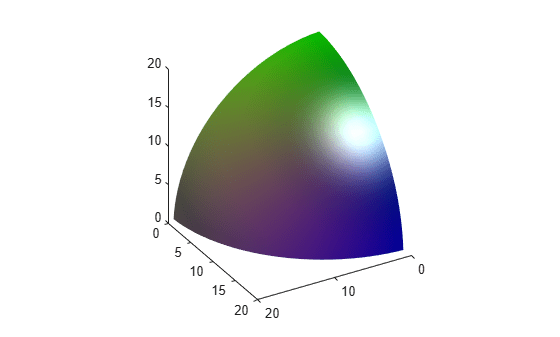Main Content

# isocolors

Calculate isosurface and patch colors

## Syntax

```nc = isocolors(X,Y,Z,C,vertices) nc = isocolors(X,Y,Z,R,G,B,vertices) nc = isocolors(C,vertices) nc = isocolors(R,G,B,vertices) nc = isocolors(...,PatchHandle) isocolors(...,PatchHandle) ```

## Description

`nc = isocolors(X,Y,Z,C,vertices)` computes the colors of isosurface (patch object) vertices (`vertices`) using color values `C`. Arrays `X`, `Y`, `Z` define the coordinates for the color data in `C` and must be monotonic vectors that represent a Cartesian, axis-aligned grid (as if produced by `meshgrid`). The colors are returned in `nc`. `C` must be 3-D (index colors).

`nc = isocolors(X,Y,Z,R,G,B,vertices)` uses `R`, `G`, `B` as the red, green, and blue color arrays (true colors).

`nc = isocolors(C,vertices)`, and `nc = isocolors(R,G,B,vertices)` assume `X`, `Y`, and `Z` are determined by the expression

`[X Y Z] = meshgrid(1:n,1:m,1:p) `

where `[m n p] = size(C)`.

`nc = isocolors(...,PatchHandle)` uses the vertices from the patch identified by `PatchHandle`.

`isocolors(...,PatchHandle)` sets the `FaceVertexCData` property of the patch specified by `PatchHandle` to the computed colors.

## Examples

collapse all

This example displays an isosurface and colors it with random data using indexed color.

```[x,y,z] = meshgrid(1:20,1:20,1:20); data = sqrt(x.^2 + y.^2 + z.^2); cdata = smooth3(rand(size(data)),'box',7); p = patch(isosurface(x,y,z,data,10)); isonormals(x,y,z,data,p) isocolors(x,y,z,cdata,p) p.FaceColor = 'interp'; p.EdgeColor = 'none'; view(150,30) daspect([1 1 1]) axis tight camlight lighting gouraud```This example displays an isosurface and colors it with true color (RGB) data.

```[x,y,z] = meshgrid(1:20,1:20,1:20); data = sqrt(x.^2 + y.^2 + z.^2); p = patch(isosurface(x,y,z,data,20)); isonormals(x,y,z,data,p) [r,g,b] = meshgrid(20:-1:1,1:20,1:20); isocolors(x,y,z,r/20,g/20,b/20,p) p.FaceColor = 'interp'; p.EdgeColor = 'none'; view(150,30) daspect([1 1 1]) camlight lighting gouraud```This example uses `isocolors` to calculate the true color data using the isosurface's (patch object's) vertices, but then returns the color data in a variable (`c`) in order to modify the values. It then explicitly sets the isosurface's FaceVertexCData to the new data (`1-c`).

```[x,y,z] = meshgrid(1:20,1:20,1:20); data = sqrt(x.^2 + y.^2 + z.^2); p = patch(isosurface(data,20)); isonormals(data,p) [r,g,b] = meshgrid(20:-1:1,1:20,1:20); c = isocolors(r/20,g/20,b/20,p); p.FaceVertexCData = 1-c; p.FaceColor = 'interp'; p.EdgeColor = 'none'; view(150,30) daspect([1 1 1]) camlight lighting gouraud```## See Also

Introduced before R2006a

Download ebook